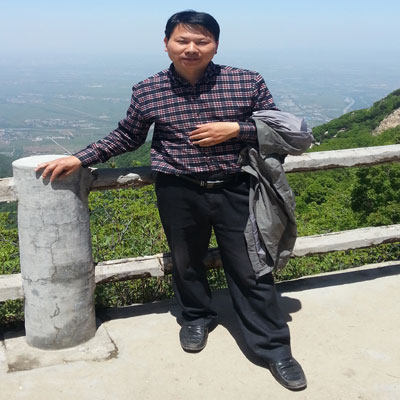# Dr. Guochang Wu

Assistant Professor
Henan University, China

Highest Degree
Ph.D. in Wavelet from Xi'an Jiaotong University, China

# Biography

Dr. Guochang Wu received M. A. at Henan University, Kaifeng, China in 2004 and the Ph.D. degree at Xian Jiaotong University, Xian, China in 2008. Since 2008, he was working as an Assistant Professor at Department of Mathematics and Information Science, Henan University of Economics and Law, Zhengzhou, China. His research interests focus on the study of Wavelet Analysis, Frame Theory, and Sampling Theory. He has recently presided over The Natural Science Foundations of Henan Province. Also, he attended the National Natural Science Foundation of China. He currently serves as technical editors for Information Technology Journal, Journal of Applied Sciences. He has served as a reviewer for many journals including Chaos, Solitons & Fractals, Computers & Mathematics with Applications, Applied Mathematics and Computation, Bulletin of the Iranian Mathematical Society, Filomat, Journal of Computational and Applied Mathematics, Mathematical Modelling and Analysis, Acta Mathematica Sinica, Information Technology Journal, Journal of Applied Sciences. He has published over 40 articles in different journals.

# Area of Interest:

Computer Sciences
100%
Information Technology
62%
Software Engineering
90%
Compressing Sensing
75%
Computational Mathematics
55%

Books
0
Chapters
0
Articles
0
Abstracts
0

# Selected Publications

1. Wu, G. and J. Qi, 2016. Construction of modified green-sch energy and its applications. Scient. Sin. Math., 46: 1127-1138, (In Chinese).
2. Wu, G., Y. Ren and B. Su, 2015. Notes on levin type inequalities of harmonic functions. Acta Math. Sin., 58: 1-11, (In Chinese).
3. Wu, G. and H. Cao, 2015. Some characterizations and application of wave packet Parseval frame. Acta Math. Sin., 58: 91-102, (In Chinese).
4. Wu, G., D. Li and H. Cao, 2014. Necessary conditions and sufficient conditions of the wave packet frames in L2(Rn). Bull. Malaysian Math. Sci. Soc., 37: 1124-1136.
5. Wu, G. and D. Li, 2014. Characterizations of the multivariate wave packet systems. Taiwanese J. Math., 18: 1389-1409.
6. Wu, G., F. Zhang and H. Cao, 2013. Some properties of R-dual sequences. Int. J. Applied Math. Statist., 50: 326-334.
7. Wu, G. and Y. Zhang, 2012. Reproducing Systems Generated by Finite Functions. Inf. Technol. J., 11: 666-672.
8. Wang, L. and G. Wu, 2012. Symmetric composite dilation multiwavelet frames. Adv. Inform. Sci. Service Sci., 4: 294-302.
9. Liu, Z., Y. Ren and G. Wu, 2012. Orthogonal Frames and Their Dual Frames in L2 (Rd). J. Inf. Comput. Sci., 9: 1329-1336.
10. Liu, Z., X. Mu and G. Wu, 2012. MRA Parseval Frame Multiwavelets in L2(Rd). Bull. Iran. Math. Soc., 38: 1021-1045.
11. Wu, G., P. Liu and Z. Cheng, 2011. Classifying Parseval Frame Multiwavelets in Higher Dimensions. J. Inf. Comput. Sci., 8: 2799-2806.
12. Li, D., G. Wu and X. Zhang, 2011. Two sufficient conditions in frequency domain for Gabor frames. Applied Math. Lett., 24: 506-511.
13. Li, D., G. Wu and X. Yang, 2011. Unified Conditions for Wavelet Frames. Georgian Math. J., 18: 761-776.
14. Zhu, X.and G. Wu, 2010. A note on some equalities for frames in Hilbert spaces. Applied Math. Lett., 23: 788-790.
15. Wu, G.C., D.F. Li and H.M. Xiao, 2010. The M-band cardinal orthogonal scaling function. Applied Math. Comput., 215: 3271-3279.
16. Wu, G., Y. Zhang and X. Yang, 2010. Sampling theory: From shannon sampling theorem to compressing sampling. Inform. Technol. J., 9: 1231-1235.
17. Wu, G. and H. Xiao, 2010. Parseval Frame Multiwavelets Associated with A-FMRA. J. Comput. Inf. Syst., 6: 1943-1950.
18. Liu, Z., G. Wu and X. Yang, 2010. Frames and sampling theorems in multiwavelet subspaces. J. Applied Math. Inform., 28: 723-737.
19. Zhu, X. and G. Wu, 2009. A Characteristic Description of Orthonormal Wavelet on Subspace L2E (R) of L2 (R). Chaos, Solitons Fractals, 41: 2484-2490.
20. Wu, G.C., Z.Q. Li and Z.X. Cheng, 2009. Construction of wavelets with composite dilations. Chaos, Solitons and Fractals, 40: 2447-2456.
21. Wu, G.C., L. Zhanwei and Y. Xiaohui, 2009. MRA parseval frame wavelets and their multipliers in L2(Rn). Math. Problems Eng., 2009: 1-17.
22. Wu, G., Y. Zhang and Z. Cheng, 2009. The cardinal orthogonal scaling function in higher dimension. Inform. Technol. J., 8: 393-397.
23. Liu, Z., G. Hu and G. Wu, 2009. Frame scaling function sets and frame wavelet sets in Rd. Chaos Solitons Fractals, 40: 2483-2490.